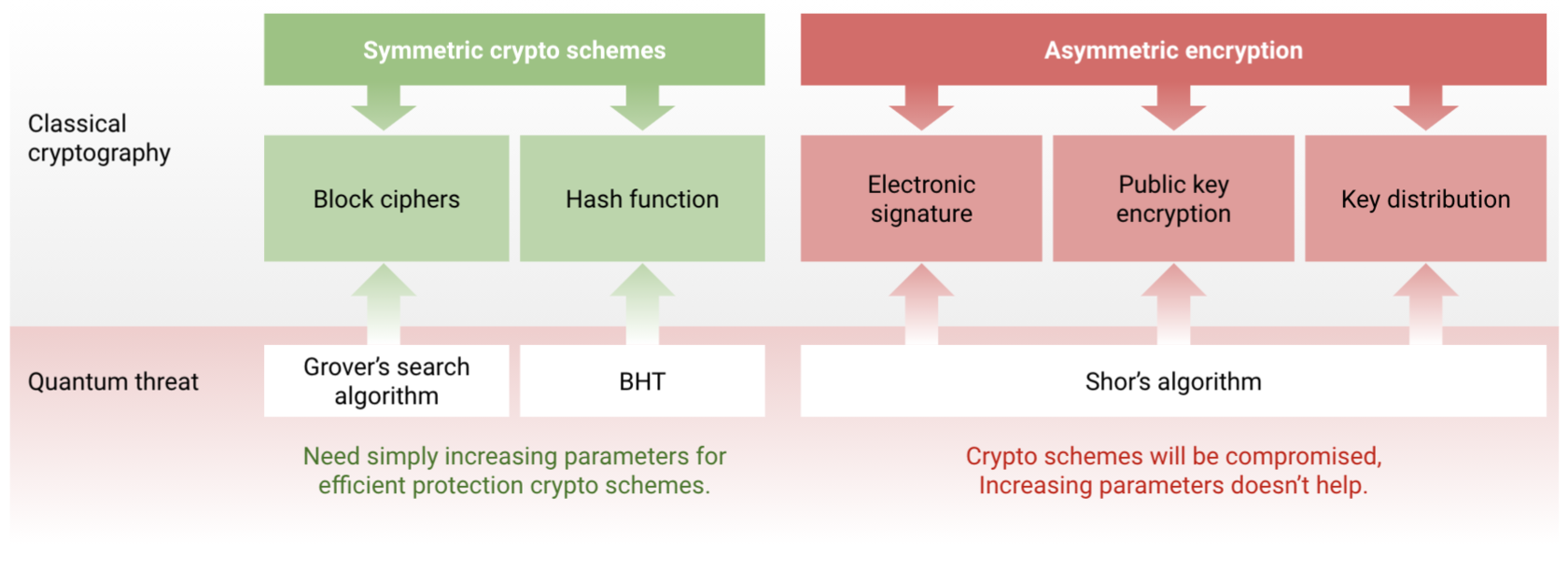Post-quantum cryptography
Synonyms: Quantum-safe algorithms, quantum-resistant algorithms
Summary

• Post-quantum cryptography comprises new cryptographic algorithms resistant to quantum computer-based attacks;
• The international cryptographic community is currently looking for the best post-quantum algorithms; this, however, does not prevent data protection specialists from implementing and piloting solutions based on already proven quantum-resistant algorithms;
• Post-quantum algorithm-based solutions for data security, which are being developed and used nowadays, do not replace traditional encryption methods but enhance them.
More on the topic

Post-quantum cryptographic algorithms are based on a special class of mathematical transformations, which are very difficult for both classical and quantum computers to invert.

Using post-quantum algorithms for data transfer and storage will improve the security of data with a lifecycle of over five years.

Over the next few years, many traditional cryptographic algorithms will become entirely obsolete. Among these algorithms are:
• Key distribution (ECDH, DH);
• Asymmetric encryption (RSA);
• Electronic signature (ECDSA, DSA).Since 2016, the US National Institute of Standards and Technology (NIST) has been testing various post-quantum algorithms to determine the best ones. The final choice will have been made by 2023. We are keeping close track of this process and provide products and services based solely on the leading and scientifically approved post-quantum algorithms.
In terms of the computational complexity theory, current public-key cryptography is based on a class of BQP (bounded-error quantum polynomial) problems. Such problems can be solved by a quantum computer in polynomial time.

Post-quantum algorithms, on the contrary, do not belong to this class: there are currently no known classical or quantum algorithms able to solve them. The mathematical problems that provide corresponding transformations include:
• problems of decoding complete linear codes;
• shortest vector problem;
• problems of finding collision/ preimage/second preimage for cryptographic hash functions.

These problems form the corresponding areas of post-quantum algorithm development:
• code-based cryptography;
• lattice-based cryptography;
• hash-based cryptography;
• etc.

How to implement post-quantum cryptography for business data protection?

Interested in using post-quantum cryptography? Explore our PQLR SDK library of quantum-resistant algorithms.

Solutions found in our library can be integrated into various types of data protection applications, including those for the protection of government information systems, trade secrets and personal data.

Not only does the library help to implement post-quantum algorithms, but it also simplifies their integration process into an existing data protection infrastructure. For this purpose, it includes a modified version of the OpenSSL library. Choosing it over the original OpenSSL ensures that applications can switch to post-quantum algorithms with minimal effort and continue using other cryptographic primitives implemented in OpenSSL.

Our library implements the following algorithms:

• key distribution algorithms and digital signature algorithms;
• algorithms based on various cryptographic schemes;
• stateless signature algorithms;
• stateful signature algorithms.

The reasons to choose post-quantum algorithms are:

1. Expert approval from international mathematical and cryptographic communities;
2. In terms of mathematical approaches, the algorithms are complementary (algorithms based on different mathematical problems are selected);
3. Theoretical validation: security proof analysis and benchmarking;
4. The algorithms have already been implemented in existing applications by third parties – industry leaders.